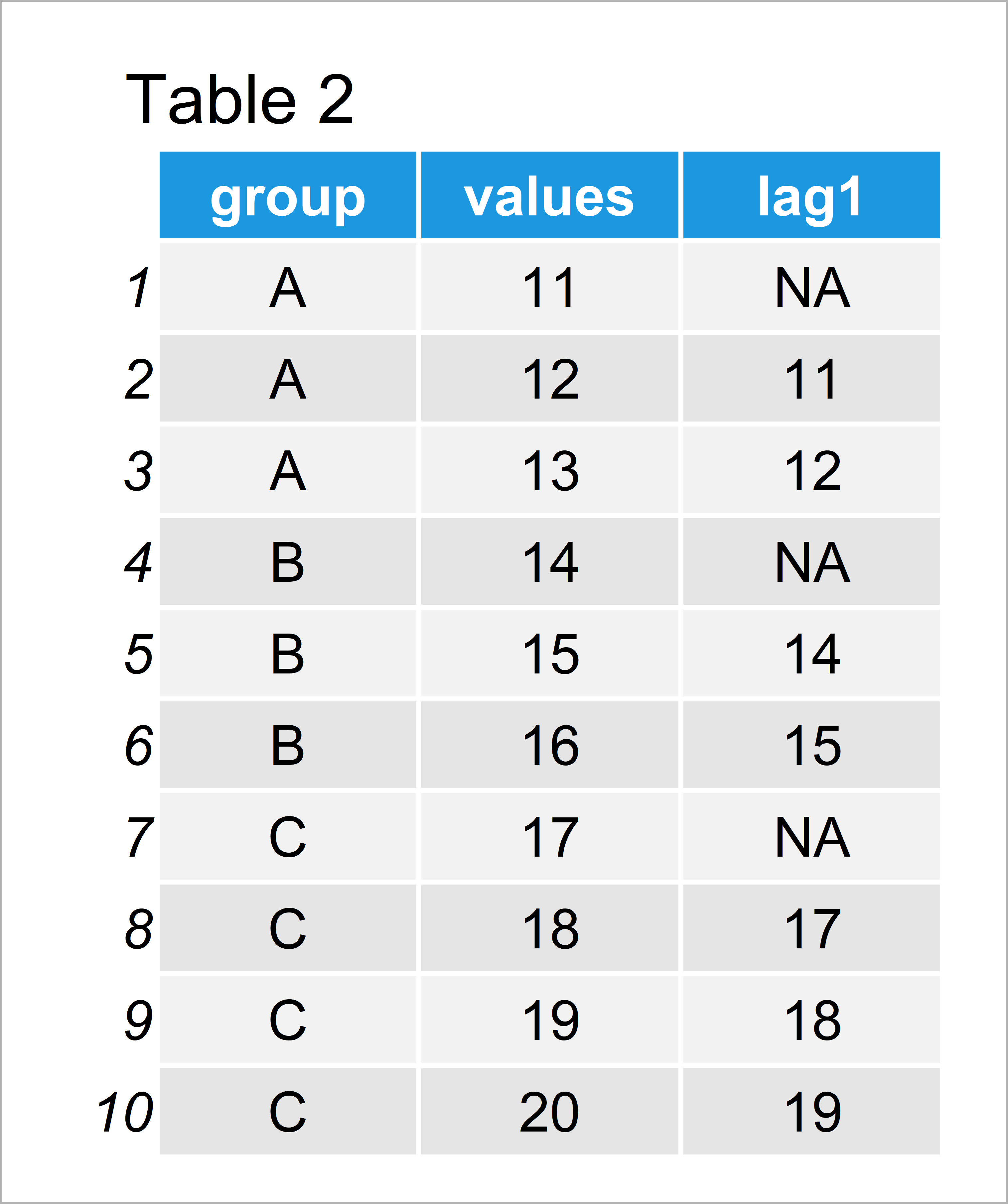# Create Lagged Variable by Group in R (Example)

In this R programming tutorial you’ll learn how to add a column with lagged values by group to a data frame.

The content is structured as follows:

You’re here for the answer, so let’s get straight to the exemplifying R code:

## Introduction of Example Data

The first step is to create some data that we can use in the examples later on:

```data <- data.frame(group = c(rep(LETTERS[1:3],    # Create example data
each = 3),
"C"),
values = 11:20)
data                                              # Print example data```Have a look at the table that has been returned after running the previous R syntax. It shows that our exemplifying data is composed of ten rows and two columns.

The variable group defines the different groups of our data and the variable values contains corresponding values.

## Example: Create Lagged Variable by Group Using dplyr Package

In this example, I’ll illustrate how to use the functions of the dplyr package to add a new column with lagged values for each group to our data frame.

First, we need to install and load the dplyr package:

```install.packages("dplyr")                         # Install dplyr package

Next, we can use the group_by, mutate, and lag functions of the dplyr package to create a new data frame containing a lagged variable by group:

```data_dplyr <- data %>%                            # Add lagged column
group_by(group) %>%
dplyr::mutate(lag1 = dplyr::lag(values, n = 1, default = NA)) %>%
as.data.frame()
data_dplyr                                        # Print updated data```In Table 2 it is shown that we have created a new data frame with a new variable called lag1.

Please note that we have specified the name of the dplyr package in front of the mutate and lag functions, because functions with the same name are also contained in other R add-on packages.

Also note that we have converted the output of the dplyr functions to the data.frame class by using the as.data.frame function. You may remove this line of code in case you prefer to return a tibble instead of a data frame.

## Video & Further Resources

I have recently released a video on my YouTube channel, which shows the R programming codes of this article. You can find the video below.

Please accept YouTube cookies to play this video. By accepting you will be accessing content from YouTube, a service provided by an external third party.If you accept this notice, your choice will be saved and the page will refresh.

Besides that, you might have a look at the related tutorials of this website.

You have learned in this tutorial how to create a lagged version of a variable by group in the R programming language. This is a very common task when dealing with time series data. In case you have additional questions, let me know in the comments section.

Subscribe to the Statistics Globe Newsletter

•Tom
January 20, 2022 4:54 pm

Thanks so much for the tutorial, it’s been really useful!

From here, how would I then go about calculating the first instance of change between the “Values” and “lag1” columns per group?

•January 20, 2022 5:55 pm

Hey Tom,

Could you specify what you mean with “first instance of change”?

Regards,
Joachim

•January 25, 2022 8:54 am

Thanks so much for the helpful tutorial!
How can I create several lagged variables in the same data?

•January 25, 2022 2:14 pm

Thank you for the kind comment, glad you found the tutorial helpful!

You may add another line of code for each additional lagged variable. For example:

```data_dplyr <- data %>%
group_by(group) %>%
dplyr::mutate(lag1 = dplyr::lag(values, n = 1, default = NA)) %>%
dplyr::mutate(lag2 = dplyr::lag(values, n = 2, default = NA)) %>%
as.data.frame()```

Regards,
Joachim

•Carmen
December 8, 2022 3:04 pm

Thanks so much for the video. Do you know how can I then calculate lagged regression with this lagged variables?

•December 13, 2022 2:59 pm